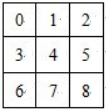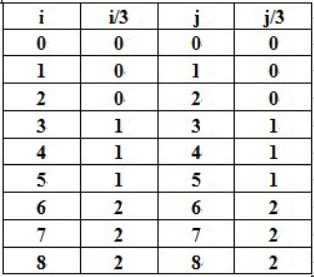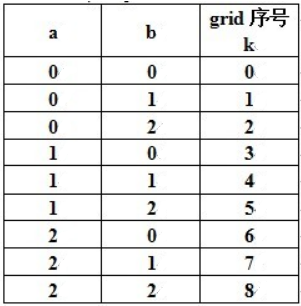# POJ 2676 - Sudoku

## 问题描述

0是待填位置，其他均为已填入的数字。

## 解题思路

DFS试探，失败则回溯

``````bool row;    //row[i][x]  标记在第i行中数字x是否出现了
bool col;    //col[j][y]  标记在第j列中数字y是否出现了
bool grid;   //grid[k][x] 标记在第k个3\*3子格中数字z是否出现了``````

row 和 col的标记比较好处理，关键是找出grid子网格的序号与 行i列j的关系，`a= i/3` , `b= j/3` ，根据九宫格的 行列 与 子网格 的 关系，我们有:## AC 源码

``````//Memory Time
//184K  422MS

#include<iostream>
using namespace std;

int map; //九宫格

bool row;    //row[i][x]  标记在第i行中数字x是否出现了
bool col;    //col[j][y]  标记在第j列中数字y是否出现了
bool grid;   //grid[k][x] 标记在第k个3*3子格中数字z是否出现了

//(这里说明的字母不代表下面程序中的变量)

bool DFS(int x,int y)
{
if(x==10)
return true;

bool flag=false;

if(map[x][y])
{
if(y==9)
flag=DFS(x+1,1);
else
flag=DFS(x,y+1);

if(flag)  //回溯
return true;
else
return false;
}
else
{

int k=3*((x-1)/3)+(y-1)/3+1;

for(int i=1;i<=9;i++)   //枚举数字1~9填空
if(!row[x][i] && !col[y][i] && !grid[k][i])
{
map[x][y]=i;

row[x][i]=true;
col[y][i]=true;
grid[k][i]=true;

if(y==9)
flag=DFS(x+1,1);
else
flag=DFS(x,y+1);

if(!flag)   //回溯，继续枚举
{
map[x][y]=0;

row[x][i]=false;
col[y][i]=false;
grid[k][i]=false;
}
else
return true;
}
}
return false;
}

int main(int i,int j)
{
int test;
cin>>test;
while(test--)
{
/*Initial*/

memset(row,false,sizeof(row));
memset(col,false,sizeof(col));
memset(grid,false,sizeof(grid));

/*Input*/

char MAP;
for(i=1;i<=9;i++)
for(j=1;j<=9;j++)
{
cin>>MAP[i][j];
map[i][j]=MAP[i][j]-'0';

if(map[i][j])
{
int k=3*((i-1)/3)+(j-1)/3+1;
row[i][ map[i][j] ]=true;
col[j][ map[i][j] ]=true;
grid[k][ map[i][j] ]=true;
}
}

/*Fill the Sudoku*/

DFS(1,1);

for(i=1;i<=9;i++)
{
for(j=1;j<=9;j++)
cout<<map[i][j];
cout<<endl;
}
}
return 0;
}``````

目录